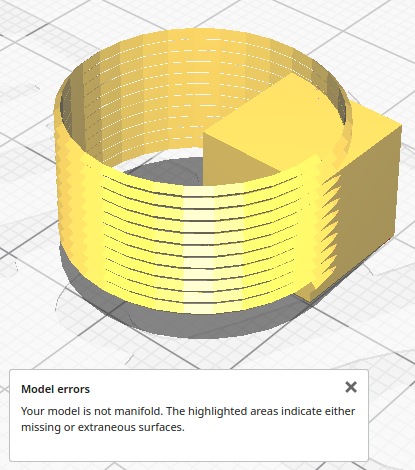# Non manifold geometry with polyhedron

• I'm trying to generate threads and I'm there for the most part. As soon as I do any boolean operations with other geometries I end up with non-manifold geometries. I'm assuming that I am either misunderstanding how to use polyhedron() or I'm defining its polygons wrong. Does anybody know what I can do?

function main() {
const r = 10;
const fn = 32;
const h = 10;

numSegments = (h+1)*fn;

const points = seq(numSegments+1).flatMap( i => threadTriangle(r,360/fn*i,i/fn-1) );

const closeFirst = [0,1,2];
const segments = seq(numSegments).flatMap( i => {
const m = i*3;
return [
[0,3,4,1].map(x => x+m),
[1,4,5,2].map(x => x+m),
[2,5,3,0].map(x => x+m)
];
})
const closeLast = [
points.length-1,
points.length-2,
points.length-3,
];

points,
polygons: [ closeFirst, ...segments, closeLast ]
});

cylinder({r:r+0.5,h,fn})
);

return union(
cylinder({r:r,h,fn})
);

}

const rad = a * Math.PI/180;
const x1 = r * Math.cos(rad);
const y1 = r * Math.sin(rad);

const x2 = (r+0.475) * Math.cos(rad);
const y2 = (r+0.475) * Math.sin(rad);

return [[x1,y1,z],[x1,y1,0.95+z],[x2,y2,0.5+z]];
}

function seq(length) {
return Array.apply(null, {length}).map(Function.call, Number);
}

• This post is deleted!

• Nevermind, I fixed everything simply by using triangles instead of polygons.

• It's completely fine until I operate on it with any other geometry.• I double checked my polygons and they are all clockwise if you are facing them.